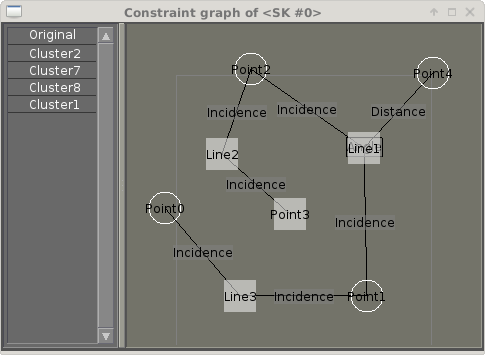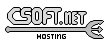Agar 1.7 Manual

AG_Graph(3)

# SYNOPSIS

```#include <agar/core.h>
#include <agar/gui.h>
```

# DESCRIPTIONThe AG_Graph widget displays a graph. A graph is composed of vertices (or nodes) and edges that connect pairs of vertices.

Edges may be either directed or undirected. Labels and colors can be associated with edges or vertices.

# INHERITANCE HIERARCHY

AG_Object(3)-> AG_Widget(3)-> AG_Graph.

# INITIALIZATION

AG_Graph * AG_GraphNew (AG_Widget *parent, Uint flags)

void AG_GraphSizeHint (AG_Graph *graph, Uint width, Uint height)

The AG_GraphNew() function allocates, initializes, and attaches a new AG_Graph widget. Acceptable flags include:
 AG_GRAPH_NO_MOVE User cannot move vertices. AG_GRAPH_NO_SELECT User cannot select vertices. AG_GRAPH_NO_MENUS Disable popup menus. AG_GRAPH_READONLY Implies AG_GRAPH_NO_MOVE, AG_GRAPH_NO_SELECT and AG_GRAPH_NO_MENUS. AG_GRAPH_HFILL Expand horizontally in parent container. AG_GRAPH_VFILL Expand vertically in parent container. AG_GRAPH_EXPAND Shorthand for AG_GRAPH_HFILL AG_GRAPH_VFILL|.

The AG_GraphSizeHint() function requests an initial widget size in pixels.

# VERTICES

AG_GraphVertex * AG_GraphVertexNew (AG_Graph *graph, void *userPtr)

AG_GraphVertex * AG_GraphVertexFind (AG_Graph *graph, void *userPtr)

void AG_GraphVertexLabel (AG_GraphVertex *vertex, const char *format, ...)

void AG_GraphVertexLabelS (AG_GraphVertex *vertex, const char *text)

void AG_GraphVertexColorLabel (AG_GraphVertex *vertex, Uint8 r, Uint8 g, Uint8 b)

void AG_GraphVertexColorBG (AG_GraphVertex *vertex, Uint8 r, Uint8 g, Uint8 b)

void AG_GraphVertexSize (AG_GraphVertex *vertex, Uint width, Uint height)

void AG_GraphVertexPosition (AG_GraphVertex *vertex, int x, int y)

The AG_GraphVertexNew() function creates a new vertex in the specified graph. userPtr is an optional user pointer to associate with the vertex.

AG_GraphVertexFind() returns the vertex matching the specified user pointer, or NULL if no match exists.

AG_GraphVertexLabel() sets the text label associated with a vertex.

AG_GraphVertexColorLabel() sets the color of the text label. AG_GraphVertexColorBG() sets the background color for the node item.

AG_GraphVertexSize() changes the default size of the node item.

AG_GraphVertexPosition() moves the node item to the given position in the view.

AG_GraphVertexPopupMenu() arranges for the given popup menu to be displayed when the user right clicks on the vertex.

# EDGES

AG_GraphEdge * AG_GraphEdgeNew (AG_Graph *graph, AG_GraphVertex *v1, AG_GraphVertex *v2, void *userPtr)

AG_GraphEdge * AG_DirectedGraphEdgeNew (AG_Graph *graph, AG_GraphVertex *v1, AG_GraphVertex *v2, void *userPtr)

AG_GraphEdge * AG_GraphEdgeFind (AG_Graph *graph, void *userPtr)

void AG_GraphEdgeLabel (AG_GraphEdge *edge, const char *format, ...)

void AG_GraphEdgeLabelS (AG_GraphEdge *edge, const char *text)

void AG_GraphEdgeColorLabel (AG_GraphEdge *edge, Uint8 r, Uint8 g, Uint8 b)

void AG_GraphEdgeColor (AG_GraphEdge *edge, Uint8 r, Uint8 g, Uint8 b)

The AG_GraphEdgeNew() function creates a new edge connecting vertices v1 and v2. If the two vertices are already connected by an edge, the function fails and returns NULL. userPtr is an optional user pointer to associated with the edge.

AG_DirectedGraphEdgeNew() identical to AG_GraphEdgeNew, but the edge is marked as being directed. Directed graph edges are assumed to point "to" v2. Directed graph edges are displayed with an arrowhead pointing towards v2 (only on systems with floating point support).

AG_GraphEdgeFind() returns the vertex matching the specified user pointer, or NULL if no match exists.

The AG_GraphEdgeLabel() sets the text label to display along the given edge.

AG_GraphEdgeColorLabel() sets the color of the text label. AG_GraphEdgeColor() sets the color of the line representing the edge. AG_GraphEdgePopupMenu() arranges for the given popup menu to be displayed when the user right clicks on the edge.

# EVENTS

The AG_Graph widget generates the following events:
 graph-vertex-selected (AG_GraphVertex *vtx) The specified vertex is now selected. graph-vertex-unselected (AG_GraphVertex *vtx) The specified vertex is no longer selected. graph-edge-selected (AG_GraphEdge *edge) The specified edge is now selected. graph-edge-unselected (AG_GraphEdge *edge) The specified edge is no longer selected.

# STRUCTURE DATA

For the AG_Graph object:
 int xOffs, yOffs Display offset in pixels. Uint nVertices Vertex count (read-only). Uint nEdges Edge count (read-only) TAILQ vertices List of AG_GraphVertex items (read-only) TAILQ edges List of AG_GraphEdge items (read-only).

For the AG_GraphVertex structure:
 int x, y Pixel coordinates of vertex in the graph. Uint w, h Bounding box for graphical representation of the vertex. void *userPtr Generic user pointer. TAILQ edges List of AG_GraphEdge(3) objects connected to this vertex.

For the AG_GraphEdge structure:
 AG_GraphVertex *v1, *v2 Vertices connected by edge void *userPtr User pointer enum ag_graph_edge_type type Either AG_GRAPH_EDGE_UNDIRECTED or AG_GRAPH_EDGE_DIRECTED to indicate an undirected or directed edge respectivelywww.libAgar.org is © 2022 Julien Nadeau Carriere <vedge@csoft.net>.